Bian, Y., Sheng, B., and Zhou, A. (2020). "An approximate solution of compact test specimen for mode I fracture test by variational approach: Applied to fibrous composite," BioRes. 15(3), 7223-7233.

#### Abstract

The present work presented an approximate solution for a compact test (CT) specimen that was employed as a standard test provided by ASTM E399-19 (2019). The variational method was employed to obtain the solution. The method used a two-step strategy to approximate the displacement response of the CT specimen. The first step was to obtain the general form of displacement solution, and then, the Rayleigh-Ritz approach was employed to modify the solution of the first step. A compliance equation of the CT specimen was obtained, and furthermore, the formula to calculate the stress intensity factor was obtained. The solution was validated by finite element (FE) model and the formula specified in ASTM E399-19 (2019). It was concluded that the calculation results of the proposed solution agreed well with the results of the FE model prediction for the ratio of initial crack length-to-ligament length, which was in the range of 0.25 to 0.35. Furthermore, compared to the results predicted by using the formula addressed in ASTM E399-19 (2019), the method proposed in the present study can achieve closer results than that of the FE model.

An Approximate Solution of Compact Test Specimen for Mode I Fracture Test by Variational Approach: Applied to Fibrous Composite

Yuling Bian,a,b,* Baolu Sheng,b and Aiping Zhou b,c

The present work presented an approximate solution for a compact test (CT) specimen that was employed as a standard test provided by ASTM E399-19 (2019). The variational method was employed to obtain the solution. The method used a two-step strategy to approximate the displacement response of the CT specimen. The first step was to obtain the general form of displacement solution, and then, the Rayleigh-Ritz approach was employed to modify the solution of the first step. A compliance equation of the CT specimen was obtained, and furthermore, the formula to calculate the stress intensity factor was obtained. The solution was validated by finite element (FE) model and the formula specified in ASTM E399-19 (2019). It was concluded that the calculation results of the proposed solution agreed well with the results of the FE model prediction for the ratio of initial crack length-to-ligament length, which was in the range of 0.25 to 0.35. Furthermore, compared to the results predicted by using the formula addressed in ASTM E399-19 (2019), the method proposed in the present study can achieve closer results than that of the FE model.

Keywords: Mode I fracture; Fracture toughness; Variational approach; Compact specimen

Contact information: a: Wuxi Vocational Institute of Commerce, 214000 Wuxi, China; School of Civil Engineering, Nanjing Forestry University, Nanjing 210037, China; b: National Engineering Research Center of Biomaterials, Nanjing Forestry University, Nanjing 210037, China; c: SouthFOR Innovations for Biomaterials and Structures, Nanjing 210037, China; *Corresponding author: bianyuling@wxic.edu.cn

INTRODUCTION

Virtually all fibrous composites are characterized with anisotropy, and in most cases they can be deemed as orthotropic material. The damage or failure of fibrous composite containing cracks can be characterized by fibrous interfacial crack propagation. To evaluate crack tolerance of orthotropic material, Sith et al. (1965) derived a formula providing the relationship of fracture toughness between isotropic and orthotropic materials. Hence the theory of linear elastic fracture mechanics (LEFM) can be adopted to evaluated fracture toughness of fibrous composites.

Linear elastic fracture mechanics (LEFM) introduced the Westergaard stress function (Unger 1995) that developed the analytical solutions for near-tip field. The singularity in near-tip field was treated by using the stress intensity factor (SIF). For brittle material, the SIF was considered as a material property that can be experimentally determined. According to the theory of LEFM, the SIF of a plane solid with a through thickness crack subjected to opening forces can be given by (Perez 2004) Eq. 1,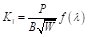(1)

where B and W are the specimen width (mm) and ligament length (mm), respectively, P is the applied opening force (N), () is the function related to the standardized crack length, =a/W is the standardized crack length (mm), and a is the crack length (mm). Equation 1 was obtained based on the Westergaad solution for the infinite plate subjected to the in-plane opening stress.

The function () serves as a modification that is strongly dependent on the geometry of the test specimen. The expressions of the modification for various geometric test specimens can be found in many references (Perez 2004; Gdouts 2005; Gross and Seelig 2011), and those of standard specimens are provided by ASTM E399-19 (2019). For a compact specimen loaded with opening force, the modification function can be given by Eq. 2,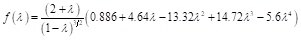(2)

Equation 2 is obtained by data fitting for numerical analyses and is validate only for a specific specimen. In fact, the theory of LEFM provides a universal method to determine the fracture toughness by differentiating the compliance of test specimen with respect to crack length. Therefore, to build the function of compliance with respect to crack length is essential to experimentally determine the fracture toughness. However, it is difficult to analytically obtain the compliance equation for many test specimens because of the complex load and boundary conditions. To date, the compliance equations in many test schemes have been obtained by a numerical model.

In the present work, the variational method was employed to derive the compliance equation of the compact test (CT) specimen, and the formula for fracture toughness calculation was further obtained by differentiating the compliance in respect to the crack length. Difficulties involved in these experiments are discussed, such as specimen size, fixture of the specimens into the testing arrangement, and stiffness of the testing equipment.

COMPLIANCE EQUATION

Figure 1(a) presents a symmetric CT specimen, with an initial crack length a, ligament length W, and width 2h, subjected to a pair of opening tensile load P. According to the symmetricity, it is reasonable to take half of the specimen into consideration, and the boundary restrictions at the symmetric axis can be assigned to be fully fixed, as illustrated in Fig. 1(b).

In the small displacement theory of elasticity displacement components, uv, of a point of the body are assumed so small that we are justified in linearizing equations governing the problem. The linearized governing equations may be summarized as follows:

Rectangular Cartesian coordinates (xy) will be employed for defining the two-dimensional space containing the body, as shown in Fig. 1(b). Thus, the displacement components in the x– and y-axis at any point (xy) in the domain of the half CT specimen can be functionally expressed as u(xy) and v(xy), respectively. The parameters u(0,y) and v(0,y) should satisfy the geometrical boundary condition: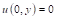and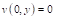(3)

For the sake of simplicity, a two-step approximation technique was employed, i.e., the first step was to obtain the general form of the displacement solution; and the second step was to determine the modification to reach a more close solution. Because the majority of the boundary satisfied the condition v(0,y)=0 and the specimen only was subjected to the opening force that was perpendicular to the y-axis, it was reasonable to approximately ignore the displacement component v(xy), and only consider the component in x direction, i.e.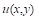.

For the sake of convenience, the authors divided the specimens into two domains, as shown in Fig. 1(b). A representative slice with a thickness of 1 mm was taken for analysis. The displacements of the two domains were u1(x,y) and u2(x,y). Then the displacements in the two domains will be given by the formulae below,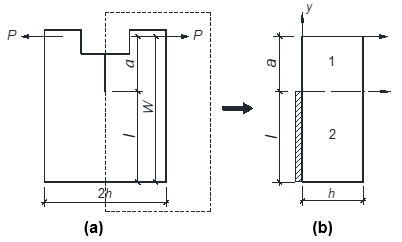Fig. 1. Modeling of the CT specimen: (a) the original specimen; (b) the equivalent half specimen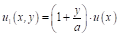(4a)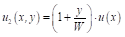(4b)

where u(x) is a function to be determined. Clearly, Eqs. 4a and 4b satisfy the continuum condition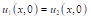. Thus, the strain in the two domains in Fig. 1(b) can be given by Eq. 5,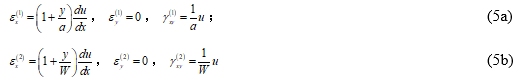where the superscript in the brackets stands for the number of domains. For plane problem, the constitutive relationships can be expressed as Eq. 6,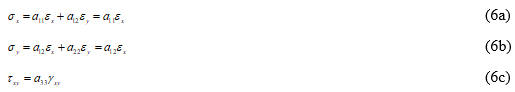where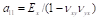,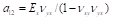,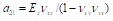,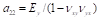, and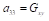;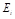,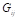, and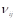(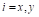,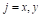) stand for the Young’s modulus, shear modulus, and Poisson’s ratio, respectively. The strain energy can be given by Eq. 7: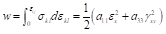(7)

Substitute Eqs. 6a, 6b, and 6c into Eq. 7 gives the strain energy for each domain as follows: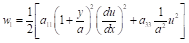(8)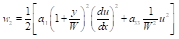(9)

According to the principle of energy conservation, one can obtain that,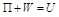(10)

where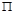is potential energy (J) and U is the strain energy (J) that is given by Eq. 11,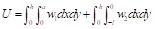(11)

and the work of the external force,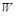, (J) can be calculated by Eq. 12: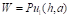(12)

Substituting Eqs. 8 and 9 into Eq. 11, and then further substituting the results into Eq. 10 lead to Eq. 13: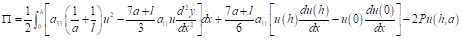(13)

Equation 13 represents the strain energy in the elastic domain 1 and 2 in total that was subjected to an opening load at point (ha).

According to the variational principle, any possible virtual displacement variation for an equilibrium system must satisfy; therefore, Eq. 14 is as follows: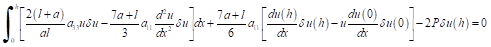(14)

Considering the boundary conditions of elastic domain, we have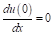, and according to Euler’s theory, we may obtain the following equations from Eq. 14: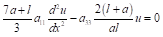(15)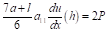(16)

For the sake of simplicity, let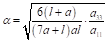，thus Eq. 15 can be expressed as: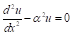(17)

The general solution of Eq. 17 can be expressed as: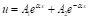(18)

Substituting the boundary condition yields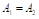at x=h and Eq. 15 into Eq. 18, after some obvious simplifications and Eq. 19 is obtained: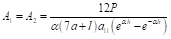(19)

Thus, the displacement function u has the form of Eq. 20: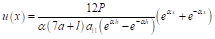(20)

Substituting Eq. 20 into Eqs. 4a and 4b gives the displacements of the domain 1 and 2 as follows: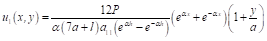(21)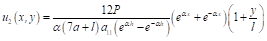(22)

Equations 21 and 22 suppose that the deformation is transverse to crack surface linear variate against coordinate y. This hypothesis is quite rough for domain 1, which is mainly subjected to bending and shearing deformation. To obtain closer displacement solution, we introduce a modification k and further express the displacement as following form based on Eqs. 21 and 22,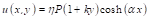(23)

where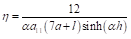, the modification k can be obtained by the Rayleigh-Ritz method.

Substituting Eq. 23 into Eq. 12 gives Eq. 24: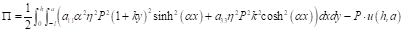(24)

According to the principle of minimum potential energy, the closest approximation k must satisfy the condition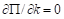, therefore Eq. 25 is as follows: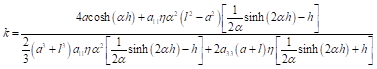(25)

It can be concluded from Equations. 23 and 25 that the deformation of the elastic body is hyperbolically variated against the coordinate y. The compliance of the CT specimen can be calculated by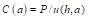. Hence, the compliance of the CT specimen can be given by Eq. 26: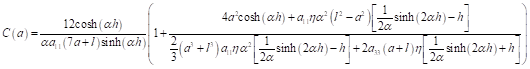(26)

FRACTURE TOUGHNESS

The fracture toughness can be obtained through differentiating of strain energy with respect to the crack length (Perez 2004; Gdouts 2005; Gross and Seelig 2011), i.e.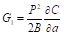, where, B is the thickness (mm) of CT specimen. Substitute Eq. 26 into this expression leads to: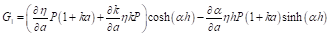(27)

The relationship of SIF and energy release rate can be given by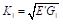(Gross and Seelig 2011). Thus, that the stress intensity can be given by,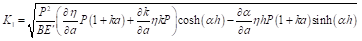(28)

where (Sith et al. 1965):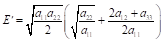(29)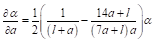(30)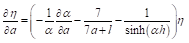(31)

For the sake of convenience, we rewrite k as the following form,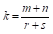(32)

where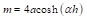,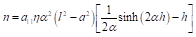,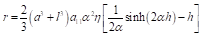, and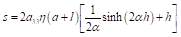; thus, we have,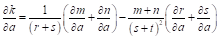(33)

where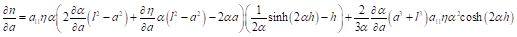(34)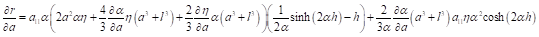(35)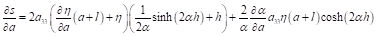(36)

NUMERICAL VALIDATION

To validate the method developed above, numerical analyses were performed through the FE Model. Parallel strand bamboo, a bamboo composites manufactured by gluing bamboo strands parallel together under controlled temperature and pressure, was selected as target material.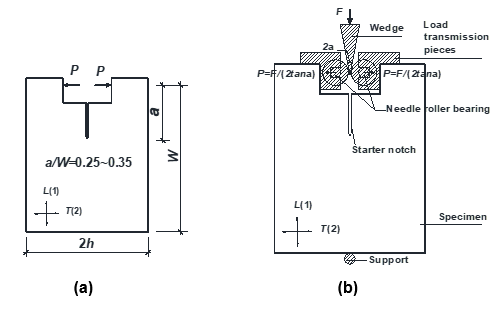Fig. 2. CT specimen: (a) configuration and dimensions of specimen (unit: mm); (b) the principle of wedge splitting test

The composite appears as a wood-like material, and the properties of bamboo composites are similar to, and usually better than wood products (Huang et al. 2013, 2015a,b). For this reason, bamboo composites are becoming a prospective alternative of wood products in construction engineering (Huang et al. 2016, 2019a; Li et al. 2017; Shen et al. 2017; Xiao et al. 2017). It has been found that the cracking or the debonding along fiber and resin interfaces is the major effect of structural damage and failure due to inherent fine cracks and microvoids in the material (Huang et al. 2018, 2019b). The fracture toughness of this material has been tested by Zhou et al. (2018) using a CT specimen as shown in Fig. 2.

The dimensions of the CT samples were determined in accordance with the test specimens tested previously by Zhou et al. (2018). As illustrated in Fig. 2(a), it was necessary to address in this study that opening load was applied by wedge splitting method. It is a modified compact test scheme proposed by Brühwiler and Wittmann (1990). The principle of the test methodology is schematically illustrated in Fig. 2(b). The CT specimen with a rectangular groove and a starter notch at the bottom of the groove were placed on a narrow support fixed on a test machine. The driving force F was transmitted from wedge to specimen via a transmission device. Therefore, the external load, P, was decomposed into the component, which perpendicular to crack surface to induce Mode-I fracture. Ignoring the friction between the wedge and bearings, the crack opening force should be P = F / (2 tan ) in case the wedge angle is 2. By comparing the loading and boundary conditions of the test specimens, it can be concluded that the displacement response perpendicular to crack surface of wedge splitting specimen was the same as that of the standard CT specimen specified in ASTM E399-19 (2019). Therefore, the modification function, as Eq. 2 illustrates, was validated for the CT specimen of wedge splitting test.

The energy release rate calculation was preformatted by the J-integral approach, and the near-tip contour integrals were implemented using ABAQUS software (Abaqus Inc., Abaqus V2018, Palo Alto, CA, USA). In the ABAQUS model (FE model), the CT specimen was treated as a plane stress problem and the material was supposed to be an orthotropic one. Longitudinal direction of the material, i.e., direction-1, was orientated in axis-y of the model; hence, the transverse direction was along axis-x, as shown in Fig. 3.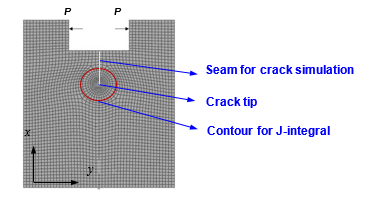Fig. 3. FEM model for J-integral

Material constants were adopted according to Table 1 according to the test results (Zhou et al. 2018). Cracks were simulated by pre-specified seams that were supposed to have the same lengths and locations as the starter notches of test specimens have. Because the seam defined an edge of a face with overlapping nodes that can be separated during analyzing process, there was no need to variational the crack faces as they are separated. In order to simulate the effect of load transmission piece, crack opening forces were applied to the real position where the test load was subjected and the distributed coupling technique in ABAQUS was adopted. The technique allowed a concentrated load acted on a reference point to produce an equivalent response of a distributed pressure. An eight-node biquadratic plane stress element (CPS8R) in the common finite element software ABAQUS was chosen to model the specimens. The contour route enclosing the crack tip for J-integral calculation was specified as shown in Fig. 3. Mesh sizes were determined in such a way in which the analysis results were minimally sensitive to the element density. The vertical component produced by the wedge was omitted due to the small angle of the wedge.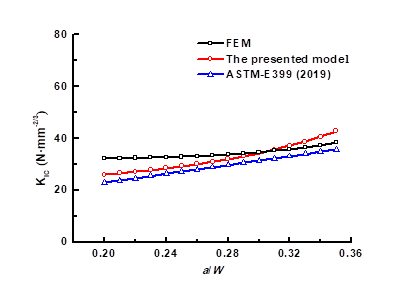Fig. 4. The KIC comparison of the FEM, formula, and standard

Table 1. The Elastic Parameters of PSB Composite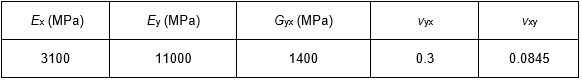Figure 4 compares the fracture toughness obtained by a different method, where the critical loads corresponding to KIC are obtained through tests (Zhou et al. 2018). It can be concluded that the calculation results agree well with the results of FE model prediction for the ratio of initial crack length-to-ligament length in the range of 0.25 to 0.35. Furthermore, the method proposed in the present study can achieve closer results than that of FE model.

CONCLUSIONS

1. An approximate solution for mode I fracture of CT specimen was derived by using a variational approach. The method used a two-step strategy to approximate the displacement response of the CT specimen. The first step was to obtain the form of displacement solution by assuming that the displacement linearly variated against the distance from crack-tip. Then a modification, k, was adopted to further approximate the displacement response. The Rayleigh-Ritz approach was employed to determine the modification.
2. To validate the solution, the critical SIF obtained by the FE model, ASTM E399-19 (2019), and the solution proposed in the present work were compared. It can be concluded that the calculation results of the proposed solution were consistent with the results of FE model prediction for the ratio of initial crack length-to-ligament length in the range of 0.25 to 0.35.
3. Furthermore, compared to the results predicted by using the formula addressed in ASTM E399-19 (2019), the method proposed in the present study can achieve closer results than that of the FE model.

ACKNOWLEDGMENTS

This work was supported by the National Science Fund of China (No. 51778299), the Priority Academic Program Development of Jiangsu Higher Education Institutions (PAPD), and the Doctorate Fellowship Foundation of Nanjing Forestry University. Yuling Bian and Baolu Sheng contributed equally to this work.

REFERENCES CITED

ASTM E399-19 (2019). “Standard test method for linear-elastic plane strain fracture toughness KIC of metallic materials,” ASTM International, West Conshohocken, PA, USA.

Brühwiler, E., and Wittmann, F. (1990). “The wedge splitting test, a new method of performing stable fracture mechanics tests,” Engineering Fracture Mechanics 35(1-3), 117-125. DOI: 10.1016/0013-7944(90)90189-N

Gdouts, E. E. (2005). Fracture Mechanics: An Introduction, 2nd Edition, Springer, Dordrecht, Netherlands.

Gross, D., and Seelig, T. (2011). Fracture Mechanics, 2nd Edition, Springer, Heidelberg, Dordrecht, Netherlands.

Huang, D., Zhou, A., and Bian, Y. (2013). “Experimental and analytical study on the nonlinear bending of parallel strand bamboo beams,” Construction and Building Materials 35, 585-592. DOI: 10.1016/j.conbuildmat.2013.03.050

Huang, D., Bian, Y., Huang, D. M., and Zhou, A. (2015a). “An ultimate-state-based-model for inelastic analysis of intermedia slenderness PSB columns under eccentric compressive load,” Construction and Building Materials 94, 306-314. DOI: 10.1016/j.conbuildmat.2015.06.059

Huang, D., Bian, Y., Zhou, A., and Sheng, B. (2015b). “Experimental study on stress-strain relationships and failure mechanisms of parallel bamboo made from Phyllostachys,” Construction and Building Materials 77, 130-138. DOI: 10.1016/j.conbuildmat.2014.12.012

Huang, D., Sheng, B., Shen, Y., and Chui, Y. H. (2018). “An analytical solution for double cantilever beam based on elastic-plastic bilinear cohesive law: Analysis for mode I fracture of fibrous composites,” Engineering Fracture Mechanics 193, 66-76. DOI: 10.1016/j.engfracmech.2018.02.019

Huang, Z., Chen, Z., Huang, D., and Zhou, A. (2016). “The ultimate load-carrying capacity and deformation of laminated bamboo hollow decks: Experimental investigation and inelastic analysis,” Construction and Building Materials 117, 190-197. DOI: 10.1016/j.conbuildmat.2016.04.115

Huang, Z., Chen, Z., Huang, D., and Chui, Y. H. (2019a). “Cyclic loading behavior of an innovative semi-rigid connection for engineered bamboo-steel hybrid frames,” Journal of Building Engineering 24, Article ID 100754. DOI: 10.1016/j.jobe.2019.100754

Huang, Z., Huang, D., Chui, Y. H., and Chen, Z. (2019b). “A bi-linear cohesive law-based model for mode II fracture analysis: Application to ENF test for unidirectional fibrous composites,” Engineering Fracture Mechanics 213, 131-141. DOI: 10.1016/j.engfracmech.2019.03.045

Li, Y., Yao, J., Li, R., Wei, Z., and Zhang, J. (2017). “Thermal and energy performances of bamboo-steel composite wall structure,” Construction and Building Materials 156, 225-237. DOI: 10.1016/j.enbuild.2017.09.083

Perez, N. (2004). Fracture Mechanics, Kluwer Academic Publishers, Boston, MA, USA.

Shen, Y., Huang, D., Zhou, A., and Hui, D. (2017). “An inelastic model for ultimate state analysis of CFRP reinforced PSB beams,” Composites Part B: Engineering 115, 266-274. DOI: 10.1016/j.compositesb.2016.09.089

Sith, G. C., Paris, P. C., and Irwin, G. R. (1965). “On cracks in rectilinearly anisotropic bodies,” International Journal of Fracture Mechanics 1(2), 189-203. DOI: 10.1007/bf00186854

Unger, D. J. (1995). Analytical Fracture Mechanics, Dover Publications, Mineola, NY, USA.

Xiao, Y., Wu, Y., Li, J., and Chen, R. Z. (2017). “An experimental study on shear strength of glubam,” Construction and Building Materials 150, 490-500. DOI: 10.1016/j.conbuildmat.2017.06.005

Zhou, A., Huang, Z., Shen, Y., Huang, D., and Xu, J. (2018). “Experimental investigation of Mode-I fracture of parallel strand bamboo composite,” BioResources 13(2), 3905-3921. DOI: 10.15376/biores.13.2.3905-3921

Article submitted: March 4, 2020; Peer review completed: June 13, 2020; Revised version received and accepted: July 24, 2020; Published: August 3, 2020.

DOI: 10.15376/biores.15.3.7223-7233